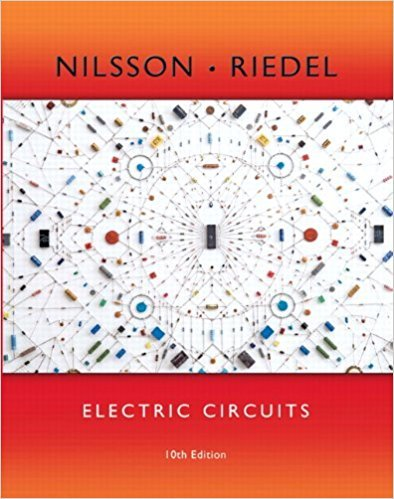×
×

# Solved: a) Replace the 2 V source in the circuit in Fig. P5.1 and calculate for each ofISBN: 9780133760033 390

## Solution for problem 5.2 Chapter 5

Electric Circuits | 10th Edition

• Textbook Solutions
• 2901 Step-by-step solutions solved by professors and subject experts
• Get 24/7 help from StudySoup virtual teaching assistantsElectric Circuits | 10th Edition

4 5 1 238 Reviews
27
0
Problem 5.2

a) Replace the 2 V source in the circuit in Fig. P5.1 and calculate for each of the following source values: 6 V, 3.5 V, 1.25 V, 2.4 V, 4.5 V, 5.4 V. b) Specify the range of voltage source values that will not cause the op amp to saturate

Step-by-Step Solution:
Step 1 of 3

l,ACrt (x()= =7f Ynr+ - r- tP) E : Y, + -.: l',Oz pt)T .;h J\. VorCu O ig.^) +N .-\z =- t 14'l, ,ltr 41 /...

Step 2 of 3

Step 3 of 3

##### ISBN: 9780133760033

This textbook survival guide was created for the textbook: Electric Circuits, edition: 10. This full solution covers the following key subjects: . This expansive textbook survival guide covers 107 chapters, and 1357 solutions. Electric Circuits was written by and is associated to the ISBN: 9780133760033. The answer to “a) Replace the 2 V source in the circuit in Fig. P5.1 and calculate for each of the following source values: 6 V, 3.5 V, 1.25 V, 2.4 V, 4.5 V, 5.4 V. b) Specify the range of voltage source values that will not cause the op amp to saturate” is broken down into a number of easy to follow steps, and 50 words. Since the solution to 5.2 from 5 chapter was answered, more than 226 students have viewed the full step-by-step answer. The full step-by-step solution to problem: 5.2 from chapter: 5 was answered by , our top Engineering and Tech solution expert on 03/13/18, 07:48PM.

Unlock Textbook Solution